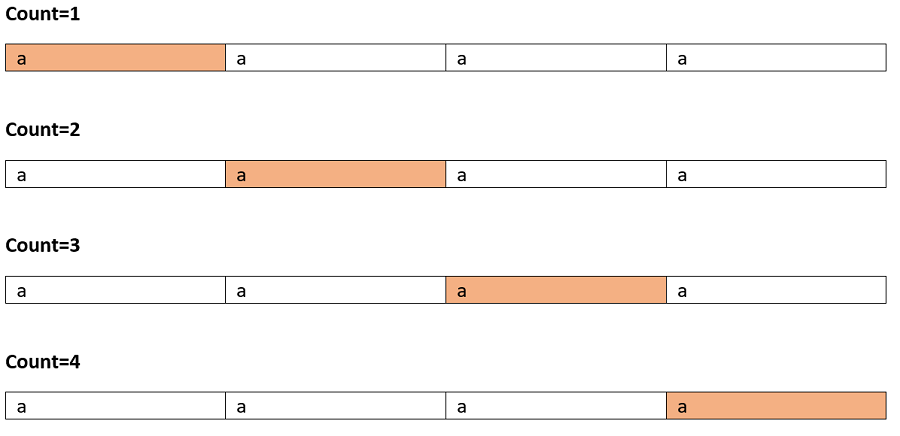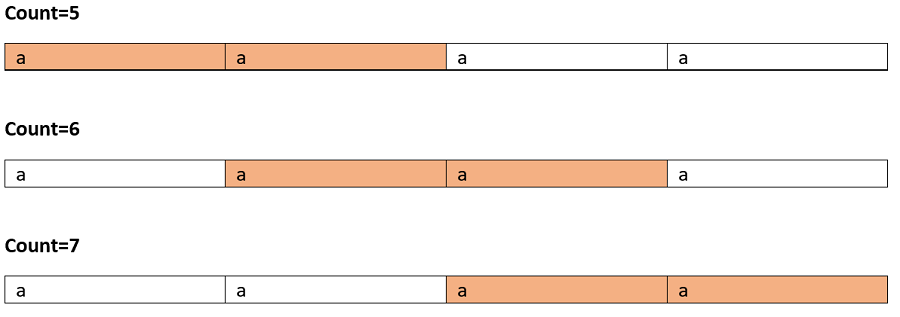# Count total number of Palindromic Substrings

Count total number of Palindromic Substrings: Here, we are going to see how to find the total number of Palindromic substrings in a string? This is a standard interview problem that can be featured in any interview coding rounds and also got featured in the amazon interview.
Submitted by Radib Kar, on June 15, 2020

Problem statement:

Given a string str, find total number of possible palindromic sub-sequences. A substring need to be consecutive such that for any xixj i<j must be valid in the parent string too. Like "incl" is a substring of "includehelp" while "iel" is not.

Input:

The first line of input contains an integer T, denoting the no of test cases then T test cases follow. Each test case contains a string str.

Output:

For each test case output will be an integer denoting the total count of palindromic substring which could be formed from the string str.

Constraints:

```1 <= T <= 100
1 <= length of string str <= 300
```

Example:

```Input:
test case:2

First test case:
Input string:
"aaaa"

Output:
Total count of palindromic substring is: 10

Second test case:
Input string:
"abaaba"

Output:
Total count of palindromic sub-sequence is: 11
```

Explanation:

Test case 1: Input: "aaaa"

The valid palindromic sub-sequences are shown below:

Marked cells are character taken in subsequence:So on...

Total 10 palindromic substrings.

Actually in this case since all the character is same each and every substring is palindrome here.

For the second test case,

```Few substrings can be,
"a"
"b"
"a"
"aba"

So on...

Total 11 such palindromic sub sequences
```

Solution approach

This can be solved by using DP bottom up approach,

1. Initialize dp[n][n] where n be the string length to 0 and a Boolean 2D array pal[n][n] to store whether the substrings are palindrome or not.
2. Fill up the base case,
Base case is that each single character is a palindrome itself. And for length of two, i.e, if adjacent characters are found to be equal then [i][i+1]=3, pal[i][i+1]=true, else if characters are different then dp[i][i+1]=2, pal[i][i+1]=false
To understand this lets think of a string like "acaa"
Here, dp=2 because there's only two palindrome possible because of "a" and "c".
Whereas for dp value will be 3 as possible substrings are "a", "a", "aa".
```for i=0 to n
// for single length characters
dp[i][i]=1;pal[i][i]=true
if(i==n-1)
break;
if(s[i]==s[i+1])
dp[i][i+1]=3;,pal[i][i+1]=true
else
dp[i][i+1]=2,pal[i][i+1]=false;
end if
end for
```
3. Compute for higher lengths,
```for len=3 to n
for start=0 to n-len
int end=start+len-1;
// start and end is matching and rest of
if(s[end]==s[start]  and pal[start+1][end-1])
// 1+subsequence from semaining part
dp[start][end]=1+dp[start+1][end]+dp[start][end-1]-dp[start+1][end-1];
Pal[start][end]=true;
else
dp[start][end]=dp[start+1][end]+dp[start][end-1]-dp[start+1][end-1];
Pal[start][end]=false;
end if
end for
end for
```
4. Final result is stored in dp[n-1];

So for higher lengths if starting and ending index is same then we recur for the remaining characters, since we have the sub-problem result stored so we computed that. In case start and end index character are different then we have added dp[start+1][end] and dp[start][end-1] that's similar to recur for leaving starting index and recur for leaving end index. But it would compute dp[start+1][end-1] twice and that why we have deducted that.

For proper understanding you can compute the table by hand for the string "aaaa" to understand how it's working.

C++ Implementation:

```#include <bits/stdc++.h>
using namespace std;

int countPS(string s)
{

int n = s.length();

if (n == 0 || n == 1)
return n;

vector<vector<int> > dp(n);
vector<vector<bool> > pal(n);

for (int i = 0; i < n; i++) {
dp[i] = vector<int>(n);
pal[i] = vector<bool>(n);
}

for (int i = 0; i < n; i++) {
dp[i][i] = 1;
pal[i][i] = true;
if (i == n - 1)
break;
if (s[i] == s[i + 1]) {
dp[i][i + 1] = 3;
pal[i][i + 1] = true;
}
else {
dp[i][i + 1] = 2;
pal[i][i + 1] = false;
}
}
for (int len = 3; len <= n; len++) {
for (int start = 0; start <= n - len; start++) {
int end = start + len - 1;
if (s[start] == s[end] && pal[start + 1][end - 1]) {
dp[start][end] = dp[start + 1][end] + dp[start][end - 1] - dp[start + 1][end - 1] + 1;
pal[start][end] = true;
}
else {
dp[start][end] = dp[start + 1][end] + dp[start][end - 1] - dp[start + 1][end - 1];
pal[start][end] = false;
}
}
}
return dp[n - 1];
}

int main()
{
int t;

cout << "Enter number of testcases\n";
cin >> t;

while (t--) {
string str;

cout << "Enter number of strings\n";
cin >> str;

cout << "Number of total palindromic substring is: " << countPS(str) << endl;
}

return 0;
}
```

Output:

```Enter number of testcases
2
Enter number of strings
aaaa
Number of total palindromic substring is: 10
Enter number of strings
abaaba
Number of total palindromic substring is: 11
```